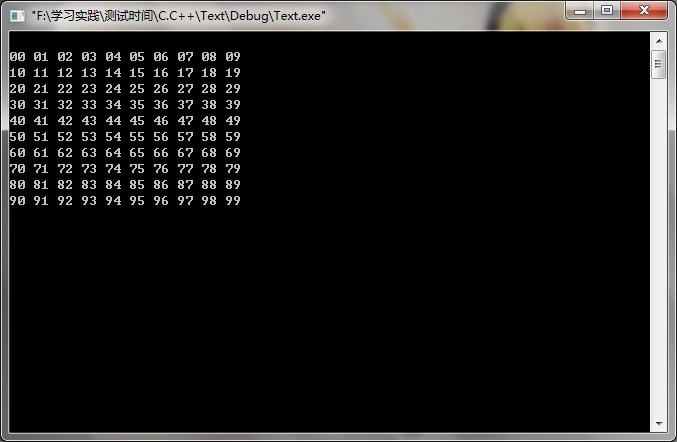# C/C++柔性数组结构成员

/*
C99中,结构中的最后一个元素允许是未知大小的数组,这就叫做柔性数组成员,但结构中的柔性数组成员前面必须至少一个其 他成员。柔性数组成员允许结构中包含一个大小可变的数组,sizeof返回的这种结构大小不包括柔性数组的内存。包含柔性数组成员的结构用malloc ()函数进行内存的动态分配,并且分配的内存应该大于结构的大小,以适应柔性数组的预期大小
C语言大全,“柔性数组成员”

*/
//看这个结构体的定义:
typedef struct st_type
{
int nCnt;
int item;
} type_a;

//有些编译器会报错无法编译可以改成:
typedef struct st_type
{
int nCnt;
int item[];
} type_a;

/*

*/
//C语言版:
type_a *p = (type_a*)malloc(sizeof(type_a) + 100*sizeof(int));

//C++语言版:
type_a *p = (type_a*)new char[sizeof(type_a) + 100*sizeof(int)];
/*

*/
//C语言版:
free(p);

//C++ 语言版:
delete [] p;

/*

*/
#include <stdio.h>
#include <conio.h>
#include <malloc.h>

//申明柔性数组结构体
typedef struct node
{
int size;
int x;
} node;

node *alloc_node(int size)
{
//动态的去申请结构体大小
node *n=(node *)malloc(sizeof(node)+sizeof(int)*size);
n->size=size;
return n;
}

int main()
{
node *n = alloc_node(100);
int i;
for (i=0; i<100; ++i)
{
n->x[i] = i;//赋值
}
for (i=0; i<100; i++)
{
if (i%10==0)
{
printf("\n");
}
printf("%.2d ",n->x[i]);//打印出结果
}
printf("\n");
free(n);
getch();
return 0;
}©️2019 CSDN 皮肤主题: 黑客帝国 设计师: 上身试试